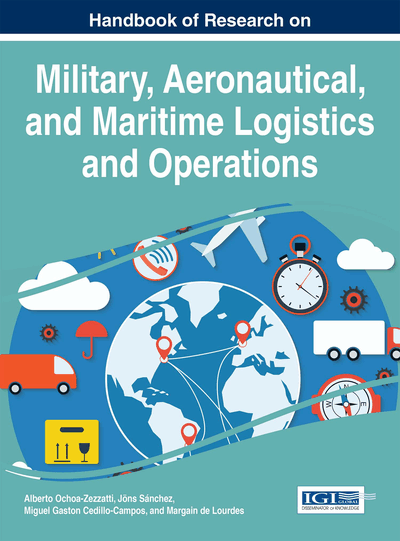# Optimization of Utility Functions in an Admissible Space of Higher Dimension

German Almanza (University Autonomous of Juarez City, Mexico), Victor M. Carrillo (University Autonomous of Juarez City, Mexico) and Cely C. Ronquillo (University Autonomous of Juarez City, Mexico)
DOI: 10.4018/978-1-4666-9779-9.ch006
Available
\$37.50
No Current Special Offers

## Abstract

S. Smale published a paper where announce a theorem which optimize a several utility functions at once (cf. Smale, 1975) using Morse Theory, this is a very abstract subject that require high skills in Differential Topology and Algebraic Topology. Our goal in this paper is announce the same theorems in terms of Calculus of Manifolds and Linear Algebra, those subjects are more reachable to engineers and economists whom are concern with maximizing functions in several variables. Moreover, the elements involved in our theorems are accessible to graduate students, also we putting forward the results we consider economically relevant.
Chapter Preview
Top

## 2. Methods

### 2.1 Utility Function

We assume an economy with l numbers of commodities and the market of each commodity have defined a unity of weight for each good, i.e. each good is quantified and is related with a real number, we will denote the real number set with. We are considering only those commodities that have in the market, that mean we use only positive real numbers. Then we denotethe orthant positive of(the real space of dimension) andto the commodity space, then there exist a homeomorphism of P with an open subset ofindeed there is a map, which is an inclusion.

The coordinateis a commodity bundle that belongs to a consumer, or the consumer wish to choose between all thecommodities. Also assume m agents in the market, we denotedto the commodity bundle of the i-agent, then existbundles foragents. The status of the economy assuming the commodities of the m agents is denoted with the point; i.e. the complete economy of the m agents is represented with a point in the commodity space of dimension. In our economic model we assume exhausted resources and those are quantified, then the next space is a natural definitions.

In the above space there are interacting the m agents and it represent the whole space of consumption or choice possibilities.

## Complete Chapter List

Search this Book:
Reset